﻿ 太阳能光伏屋顶对城市热环境及能源供需影响的模拟
 气象学报2020, Vol. 78Issue (2): 301-316PDF
http://dx.doi.org/10.11676/qxxb2020.015

0

#### 文章信息

HAO Xiaolong, WANG Yongwei, HU Ning, CHEN Weidong. 2020.

Simulation of the influence of solar photovoltaic roof on urban thermal environment and energy supply and demand

Acta Meteorologica Sinica, 78(2): 301-316.
http://dx.doi.org/10.11676/qxxb2020.015

### 文章历史

2019-07-13 收稿
2019-11-11 改回

1. 南京信息工程大学大气物理学院，南京，210044;
2. 南京信息工程大学大气环境中心，南京，210044;
3. 国家电网电力科学研究院，南京，210003

Simulation of the influence of solar photovoltaic roof on urban thermal environment and energy supply and demand
HAO Xiaolong1,2, WANG Yongwei1,2, HU Ning1,2 , CHEN Weidong3
1. School of Atmospheric Physics，Nanjing University of Information Science and Technology，Nanjing 210044，China;
2. Yale-NUIST Center on Atmospheric Environment，International Joint Laboratory on Climate and Environment Change (ILCEC)，Nanjing University of Information Science and Technology，Nanjing 210044，China;
3. State Grid Electric Power Research Institute，Nanjing 210003，China
Abstract: The installation of solar roofs is expected to alleviate to a certain extent the energy crisis caused by urbanization and the destruction of urban thermal environment. The heat transfer model of solar panels is introduced into the WRF multi-layer urban canopy scheme, and the effects of solar roofs in two installation forms (attached roof and scaffolding roof) on urban thermal environment and energy consumption balance are simulated online. A typical heat wave weather is selected for the present study. The results show that: (1) Installation of the attached solar roof can reduce 2 m temperature by 0.29°C during the daytime, and the cooling effect is better than that of the scaffolding roof, but the decrease of temperature at the nighttime is small. The scaffolding roof cools down to 0.23°C during the daytime, and the effect of cooling at the nighttime is significant. Compared with the ordinary roof, the temperature is lowered by 0.60°C. (2) The solar roof can really cool down the urban area during the daytime, but it will also weaken the turbulence, inhibit the development of the daytime boundary layer and reduce the height of the boundary layer. In the case of strong turbulence development, the weakening effect of solar roofs is more obvious. (3) In addition to impacts on urban meteorology, solar roofs can make important contributions to energy supply. From the results, the power generated by solar panels can afford 54.5% of the energy consumption by air conditioners in commercial areas during the study period.
Key words: Solar roof    Urban canopy scheme    Urban thermal environment    Energy
1 引　言

2 模式及相关数据 2.1 研究区域及天气背景

2017年夏季，中国受到大范围持续高温天气影响，江苏地区电网用电负荷突破往年极值（王国复等，2018）。选取江苏省2017年7月21—27日一次持续的高温天气过程作为研究时段。从气温高于30°C及35°C的站点数占总站点数的比例（表1）可以看出，江苏省大部分区域受到高温控制。

 21日 22日 23日 24日 25日 26日 27日 ＞35°C 0.88 0.99 0.99 1 0.67 0.64 0.93 ＞30°C 1 1 1 1 0.96 0.98 1
2.2 模式及算例图 1  （a） 嵌套区域 （方框） 和地形高度 （色阶，单位：m） 及 （b） 最内层土地利用类型 （数字） 分布 Fig. 1  （a） Nested simulation areas （black rectangles indicate D01，D02，D03） and terrain height （shaded，unit：m）；（b） land-use types （number） for the inner-most domain D03

 低密度住宅区 高密度住宅区 商业区 高度（m） 占比（%） 高度（m） 占比（%） 高度（m） 占比（%） 6 63 6 28 6 13 9 17 9 15 9 10 12 1 12 5 12 6 15 6 15 25 15 24 18 12 18 20 18 24 21 1 21 3 21 8 24 3 24 4 27 1 27 4 30 3 33 2 36 1 39 1

2.3 WRF模式中太阳能屋顶热平衡方案的引入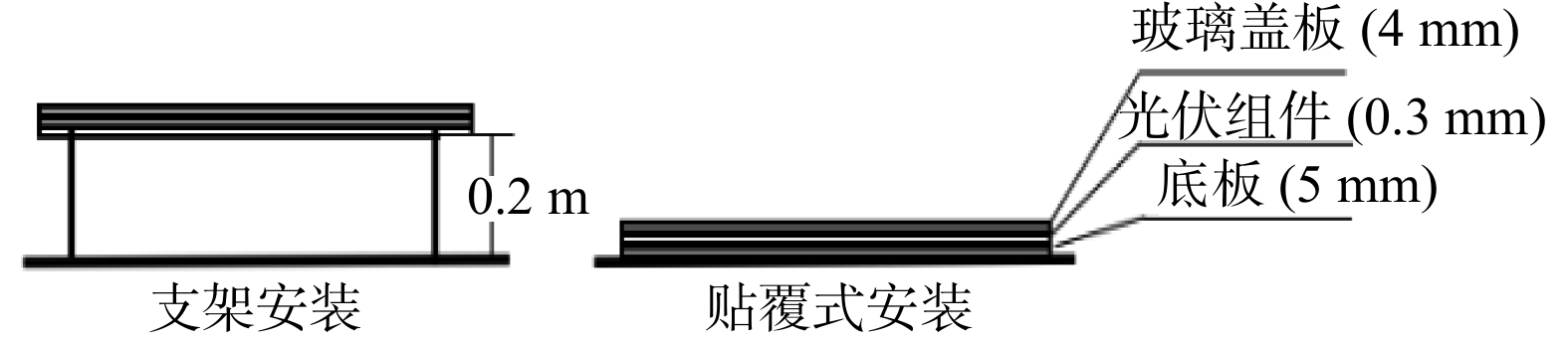图 2  屋顶安装方式示意 Fig. 2  Roof installation methods

 导热系数（W/（m·K）） 密度（kg/m3） 比热容（J/（kg·K）） 厚度（mm） 玻璃板 1.04 2500 835 4 光伏组件 150 2330 950 0.3 底板 0.14 1475 1130 5
2.3.1 屋顶有通风流道的光伏板支架热平衡计算方案

 $\begin{split}&{R_{\rm s}} \times {a_1}(1 - r) + \varepsilon \left[ {{R_{\rm l}} - \sigma {{(T_1^{{{n - 1}}})}^4}} \right] - {H_1} - {h_{12}} \times \\&\quad(T_1^{{n}} - T_2^n){\rm{ - }}\frac{{{\rho _1}{c_{{\rm p}1}}\Delta {x_1}}}{{{\rm d}t}}(T_1^{{n}} - T_1^{{{n - 1}}}) = 0\end{split}$ (1)
 $\begin{split}&{R_{\rm s}} \times {a_2}(1 - {a_1})(1 - r) - E + {h_{12}} \times (T_1^{{n}} - T_2^n) - {h_{23}} \times\\&\quad (T_2^{{n}} - T_3^n){\rm{ - }}\frac{{{\rho _2}{c_{{\rm p}2}}\Delta {x_2}}}{{{\rm d}t}}(T_2^{{n}} - T_2^{{{n - 1}}}) = 0\end{split}$ (2)
 $\begin{split}&{h_{23}} \times (T_2^{{n}} - T_3^n) + {h_{3{\rm a}}} \times (T_{\rm a}^{{n}} - T_3^n) + \varepsilon {}_1\sigma {(T_{\rm{r}}^{{{n - 1}}})^4} -\\&\quad {\varepsilon _2}\sigma {(T_3^{{{n - 1}}})^4}{\rm{ - }}\frac{{{\rho _3}{c_{{\rm p}3}}\Delta {x_3}}}{{{\rm d}t}}(T_3^{{n}} - T_3^{{{n - 1}}}) = 0\end{split}$ (3)
 $\begin{split}&{h_{3{\rm a}}} \times (T_3^{{n}} - T_{\rm a}^n) + {h_{\rm{ar}}} \times (T_{{\rm r}1}^{{n}} - T_{\rm a}^n) - \rho S{U'}{c_{\rm{pa}}} \times\\&\quad (T_{{\rm{out}}}^{{n}} - {T_{in}^n}) - \frac{{{\rho _{\rm a}}{c_{\rm{pa}}}\Delta {x_{\rm a}}}}{{{\rm d}t}}(T_{\rm a}^{{n}} - T_{\rm a}^{{{n - 1}}}) = 0\end{split}$ (4)
 $\begin{split}&{h_{\rm{ar}}} \times (T_{\rm a}^{{n}} - T_{{\rm r}1}^n) + {h_{{\rm r}12}} \times (T_{{\rm r}2}^{{n}} - T_{{\rm r}1}^n) + {\varepsilon _2}\sigma {(T_3^{{{n - 1}}})^4} -\\&\quad {\varepsilon _3}\sigma {(T_{{\rm r}1}^{{{n - 1}}})^4} - \frac{{{\rho _{{\rm r}1}}{c_{{\rm{pr}}1}}\Delta {x_{{\rm r}1}}}}{{{\rm d}t}}(T_{{\rm r}1}^{{n}} - T_{{\rm r}1}^{{{n - 1}}}) = 0\end{split}$ (5)

 参数 参数说明 参数 参数说明 ${H_{\rm{1}}}$ 光伏板表面的感热通量（W/m2） $\Delta {x_1}$ 玻璃板厚度（m） ${R_{\rm s}}$ 向下的太阳短波辐射（W/m2） $\Delta {x_2}$ 光伏组件厚度（m） ${R_{\rm l}}$ 向下的大气长波辐射（W/m2） $\Delta {x_3}$ 底板厚度（m） $r$ 玻璃板反射率 $\Delta {x_{\rm a}}$ 通风夹层空气层厚度（m） ${\rm{\varepsilon }}$ 玻璃板发射率 $\Delta {x_{{\rm r}1}}$ 屋顶第一层厚度（m） ${{\rm{\varepsilon }}_1}$ 底板发射率 ${h_{12}}$ 玻璃板与光伏组件传热系数（W/（m·K）） ${\varepsilon _2}$ 屋顶发射率 ${h_{23}}$ 光伏组件与底板传热系数（W/（m·K）） $E$ 发电量（W/m2） ${h_{3{\rm a}}}$ 底板与空气对流交换系数（W/（m·K）） ${{\rm{\rho }}_1}$ 玻璃板密度（kg/m3） ${h_{\rm{ar}}}$ 屋顶与空气对流交换系数（W/（m·K）） ${{\rm{\rho }}_2}$ 光伏组件密度（kg/m3） ${T_1}$ 玻璃板温度（K） ${{\rm{\rho }}_3}$ 底板密度 （kg/m3） ${T_2}$ 光伏电池温度（K） ${{\rm{\rho }}_a}$ 空气密度 （kg/m3） ${T_3}$ 底板温度（K） ${{\rm{\rho }}_{{\rm r}1}}$ 屋顶第一层密度（kg/m3） ${T_{\rm a}}$ 夹层气温（K） ${c_{{\rm p}1}}$ 玻璃板比热容（J/（kg·K）） ${T_{\rm{in}}}$ 进口气温（K） ${c_{{\rm p}2}}$ 光伏组件比热容（J/（kg·K）） ${T_{\rm{out}}}$ 出口气温（K） ${c_{{\rm p}3}}$ 底板比热容（J/（kg·K）） ${T^n}$ n时刻温度（K） ${c_{\rm{pa}}}$ 空气比热容（J/（kg·K）） ${c_{{\rm{pr}}1}}$ 屋顶第一层比热容（J/（kg·K）） ${a_1}$ 玻璃板吸收率 ${a_2}$ 光伏组件吸收率

 $E = {\rm{\varepsilon }}R_{\rm s}{a_2}(1 - {a_1})(1 - r)\min [1;1 - 0.005({T_2} - 298.15)]{\rm{ }}$ (6)

2.3.2 光伏屋顶与WRF/BEP/BEM方案的耦合

3 结果分析 3.1 模拟效果检验

 ${\rm{IA}}{\rm{ = }}1{\rm{ - }}\frac{{\displaystyle\sum\limits_{i = 1}^N {{{{\varphi _i}}^2}} }}{{\displaystyle\sum\limits_{i = 1}^N {(\left| {{p_i} - \bar o} \right| + } \left| {{o_i} - \bar o} \right|{)^2}}}{\rm{ }}$ (7)
 ${\rm{SD}}\_{\rm O} = {\left(\frac{1}{{N - 1}}\displaystyle\sum\limits_{i = 1}^N ({o_i} - \bar o)^2\right)}^{{1/2}}$ (8)
 ${\rm{SD}}\_{\rm P} = {\left(\frac{1}{{N - 1}}\displaystyle\sum\limits_{i = 1}^N ({p_i} - \bar p)^2\right)}^{{1/2}}$ (9)
 ${\rm{RMSE}} = {\left[\frac{1}{N}\displaystyle\sum\limits_{i = 1}^N {{{\varphi _i}}^2} \right]}^{1/2}$ (10)图 3  各气象要素 （2 m气温（a—d）、2 m相对湿度（e—h）、10 m风速（i—l）） 模拟与观测值时间序列 （a、e、i. 低密度住宅区，b、f、j. 高密度住宅区，c、g、k. 商业区，d、h、l. 郊区） Fig. 3  Comparisons of simulated and observed 2 m air temperature (a-d)，2 m relative humidity (e-h)，10 m wind speed (i-l) （a，e，i. low-density residential areas；b，f，j. high-density residential areas；c，g，k. business district；d，h，l. suburban areas）图 4  江苏省7月24日2 m气温模拟 （色阶） 与观测 （图中数字） 水平分布 （a. 02时，b. 08时，c. 14时，d. 20时） Fig. 4  Distributions of simulated （shaded） and observed （the numbers in the figures） 2 m temperature in Jiangsu Province on 24 July （a. 02:00 BT，b. 08:00 BT，c. 14:00 BT，d. 20:00 BT）

 IA RMSE SD_O SD_P 低密度住宅区 T2m（°C） 0.97 1.09 3.03 3.52 RH2m（%） 0.88 10.24 14.55 13.67 W10m（m/s） 0.71 0.95 0.70 0.80 高密度住宅区 T2m（°C） 0.97 1.02 3.12 3.44 RH2m（%） 0.86 11.03 14.16 12.90 W10m（m/s） 0.69 0.97 0.67 0.80 商业区 T2m（°C） 0.98 0.83 3.26 3.43 RH2m（%） 0.87 10.56 15.38 12.75 W10m（m/s） 0.90 0.49 0.72 0.90 郊区 T2m（°C） 0.96 1.36 3.03 3.90 RH2m（%） 0.92 7.63 13.61 13.21 W10m（m/s） 0.64 1.12 0.68 0.77

Zhang等（2017）模拟了2017年长三角地区的一次高温过程，其结果表明，对于湿度的模拟，城市和郊区站点的RSME分别为7.8%、5.5%。本次模拟结果显示，城市模拟结果偏差，其中高密度住宅区的RSME达到11.03%。但从吻合系数来看，各区都超过0.85，SD_O与SD_P的比值也较小。对风速的模拟存在高估现象，除了商业区的吻合系数达到0.9，其他区都低于0.75。低密度区、高密度区、郊区的均方根误差分别达到0.95、0.97、1.12 m/s，差别较大。WRF模式对于风速的模拟不够准确，主要原因是模式分辨率不够高，很难准确地描述出由于建筑物、植被以及地形起伏等原因对于局地流场的影响。

3.2 太阳能屋顶的设置对近地层2 m气温的影响图 5  2017年7月21—27日光伏屋顶 （a、b. 支架式，c、d. 贴覆式） 与对照算例中2 m气温 （单位：°C） 的差值 （a、c．06—18时平均降温幅度，b、d. 19—05时平均降温幅度） Fig. 5  Mean differences （unit：°C ）in 2 m air temperature between results of photovoltaic roofs （a，b. the bracket roof，c，d. the attached roof） and control run during 21—27 July 2017 （a，c. average cooling rates during 06:00-18:00 BT，b，d. average cooling rates during 19:00-05:00 BT）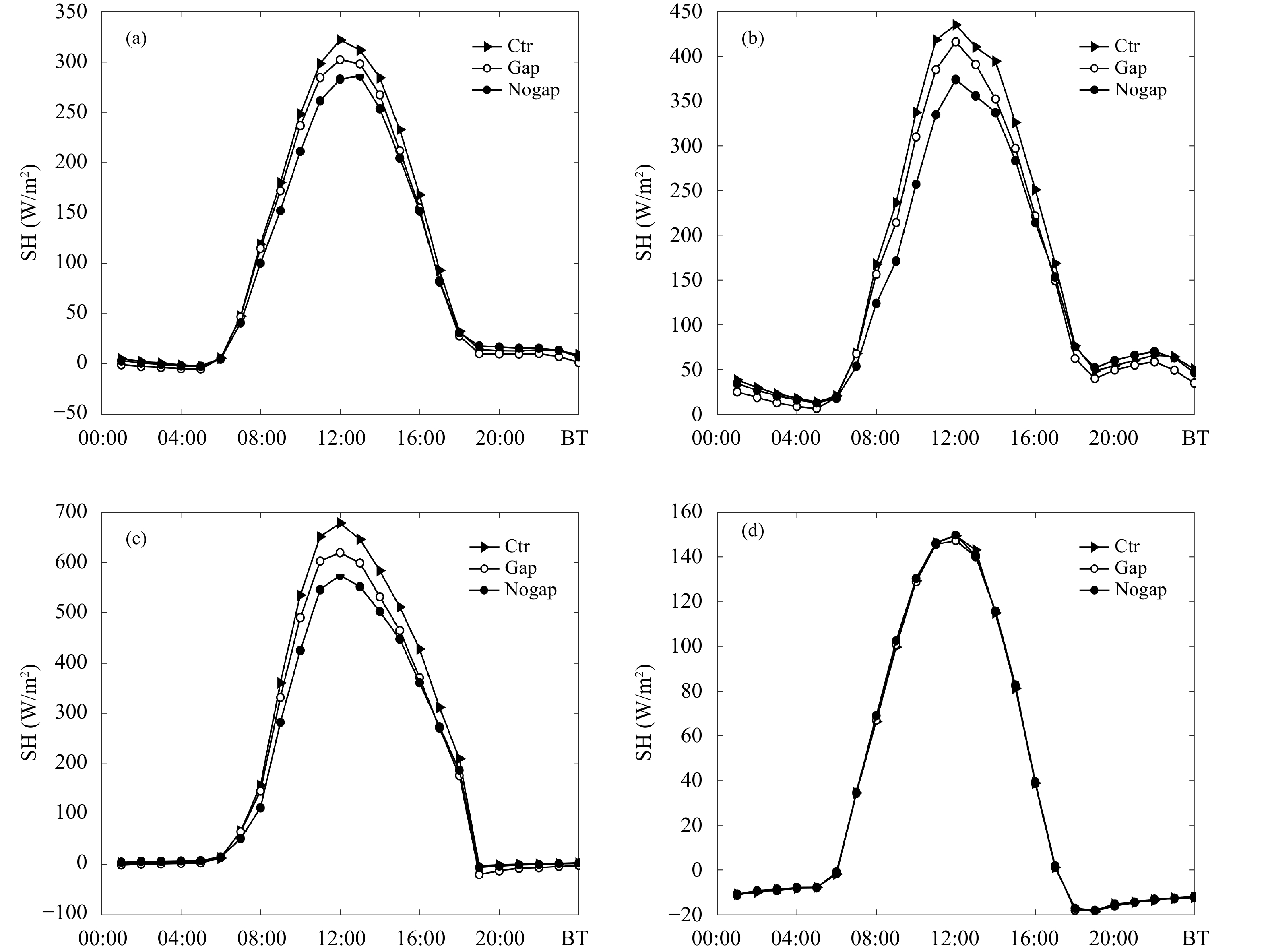图 6  对照算例及两种光伏屋顶感热通量的平均日变化特征 （a. 低密度住宅区，b. 高密度住宅区，c. 商业区，d. 郊区） Fig. 6  Diurnal variation characteristics of average sensible heat flux for the two types of photovoltaic roof （a. low-density residential areas，b. high-density residential areas，c. commercial areas，d. suburbs）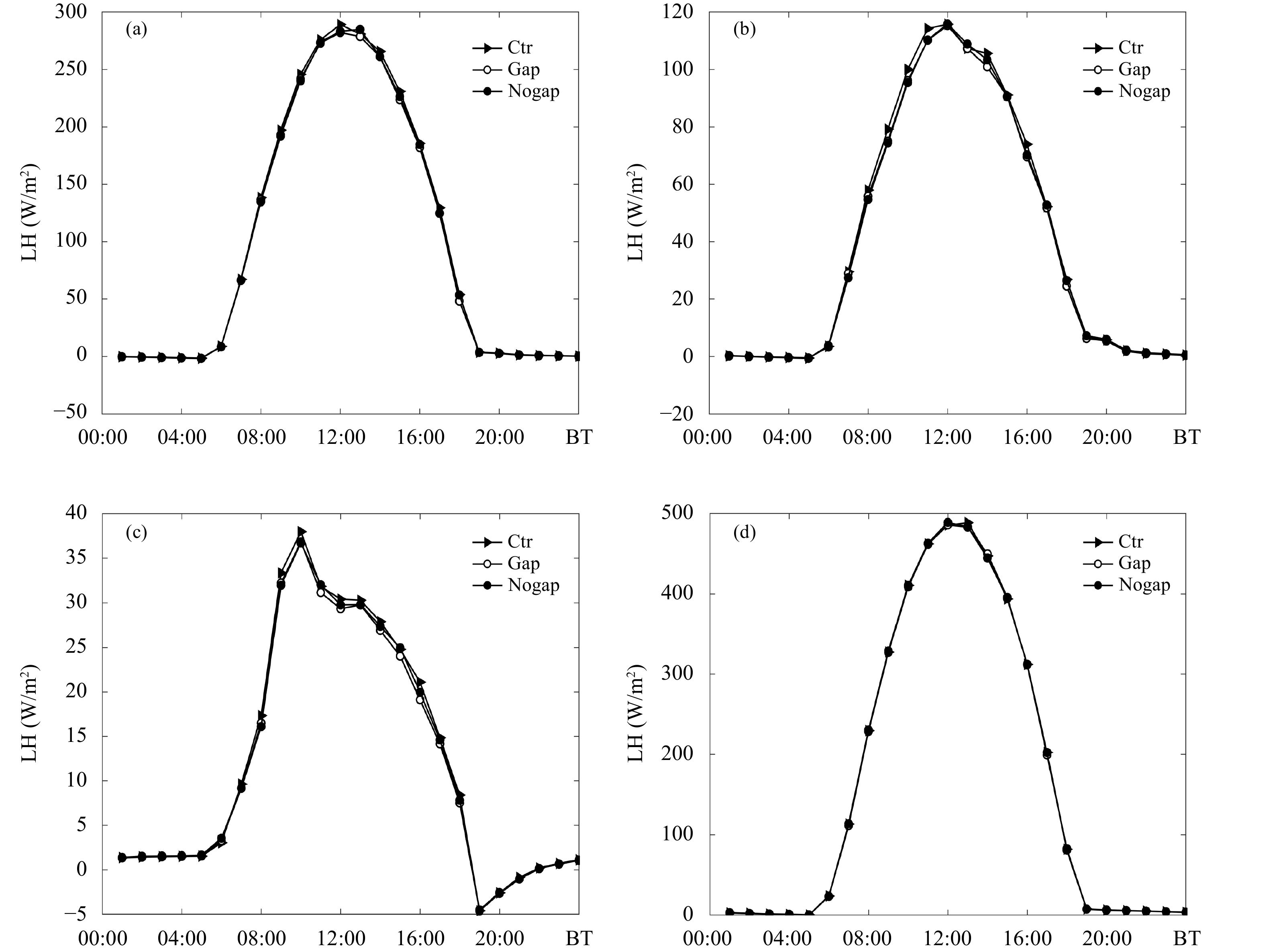图 7  对照算例及两种光伏屋顶潜热通量的平均日变化特征 （说明同图6） Fig. 7  Diurnal variation characteristics of average latent heat flux for the two types of photovoltaic roof （illustration same as Fig. 6）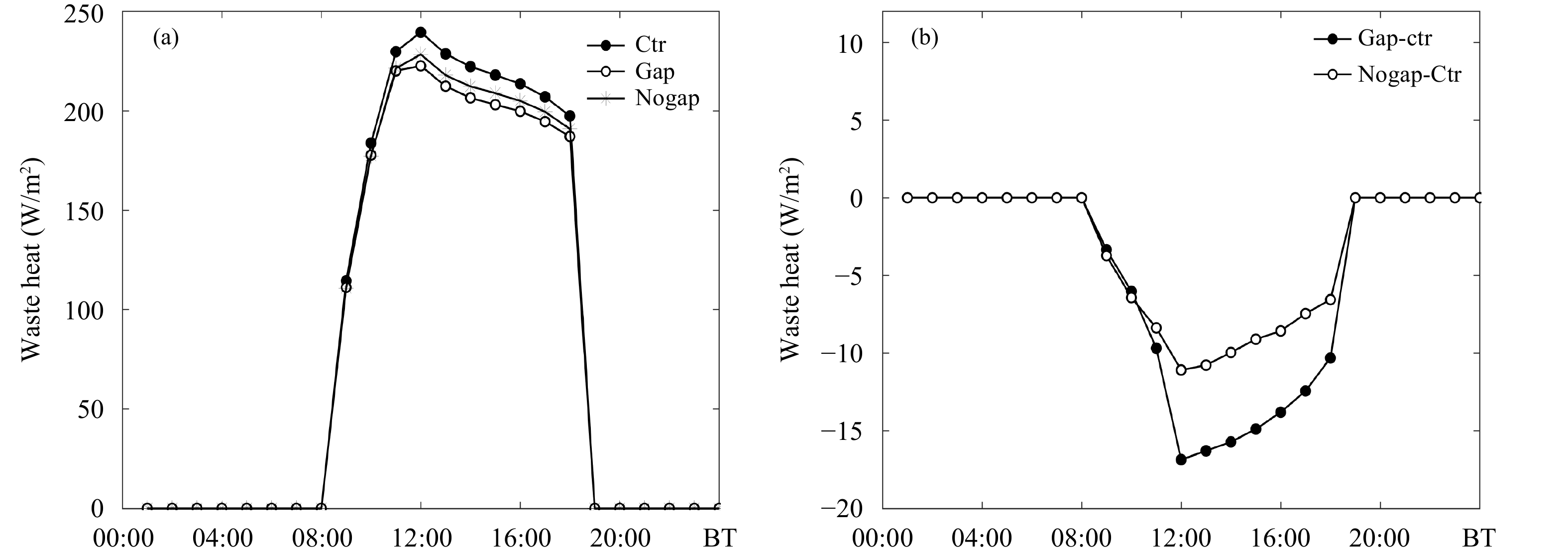图 8  使用光伏屋顶前后建筑废热排放随时间的变化 （a. 各屋顶的废热排放，b. 光伏屋顶与普通屋顶差值） Fig. 8  Waste heat from construction （a. waste heat discharge from each type of roof，b. the difference between photovoltaic roof and ordinary roof）
3.3 对边界层湍流交换的影响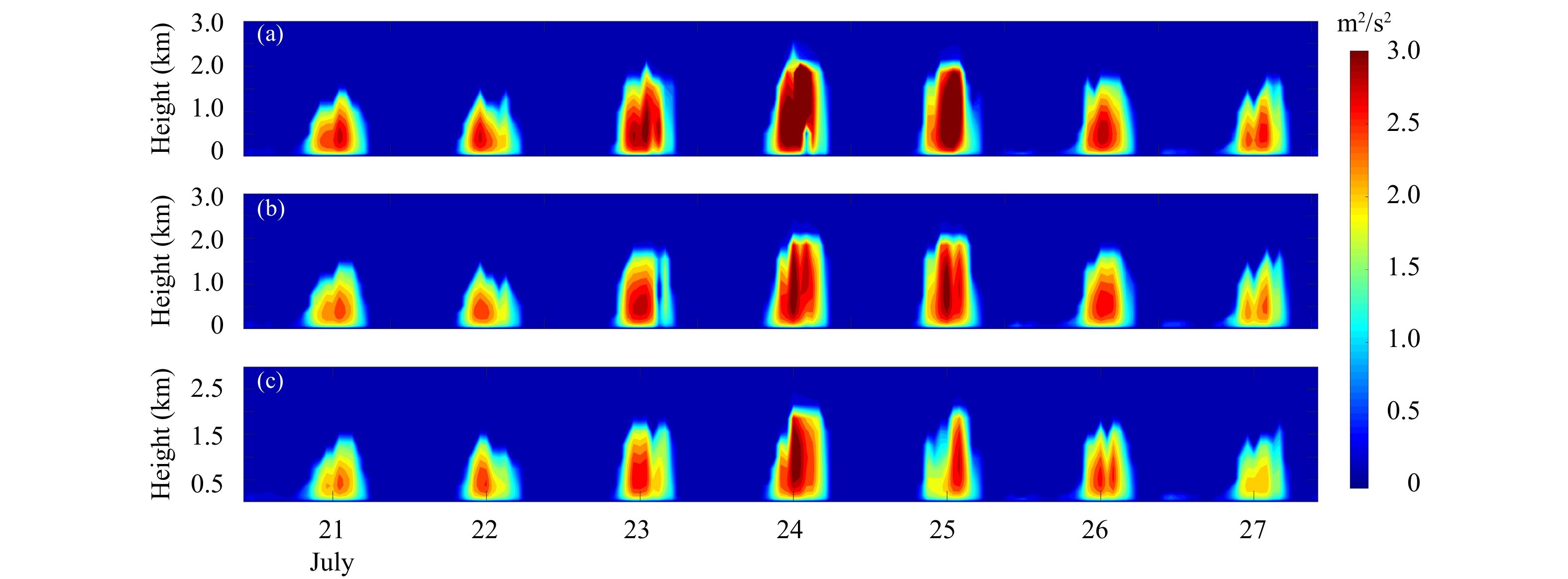图 9  南京站各屋顶湍流动能的垂直分布 （a. 普通屋顶，b. 支架式安装，c. 贴覆式安装） Fig. 9  Vertical distributions of turbulence energy for various types of roof in Nanjing Station （a. normal roof，b. scaffolding roof，c. attached roof）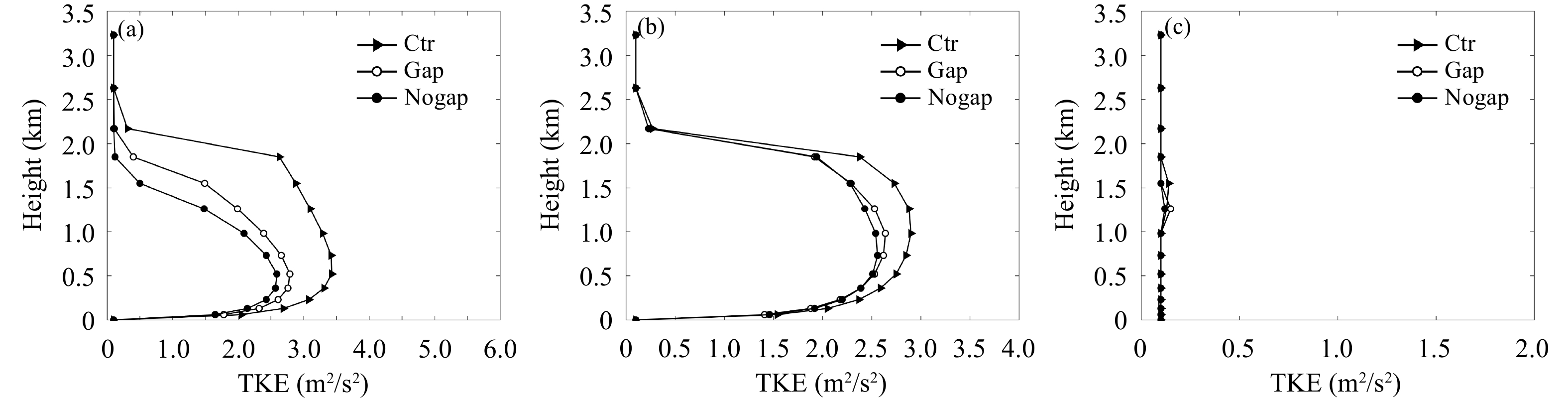图 10  7月24日不同时段湍流动能随高度变化 （a. 14时，b. 18时，c. 22时） Fig. 10  Variations of turbulent energy with altitude at different times on July 24 （a. 14:00 BT，b. 18:00 BT，c. 22:00 BT）
3.4 对能源消耗与收支的影响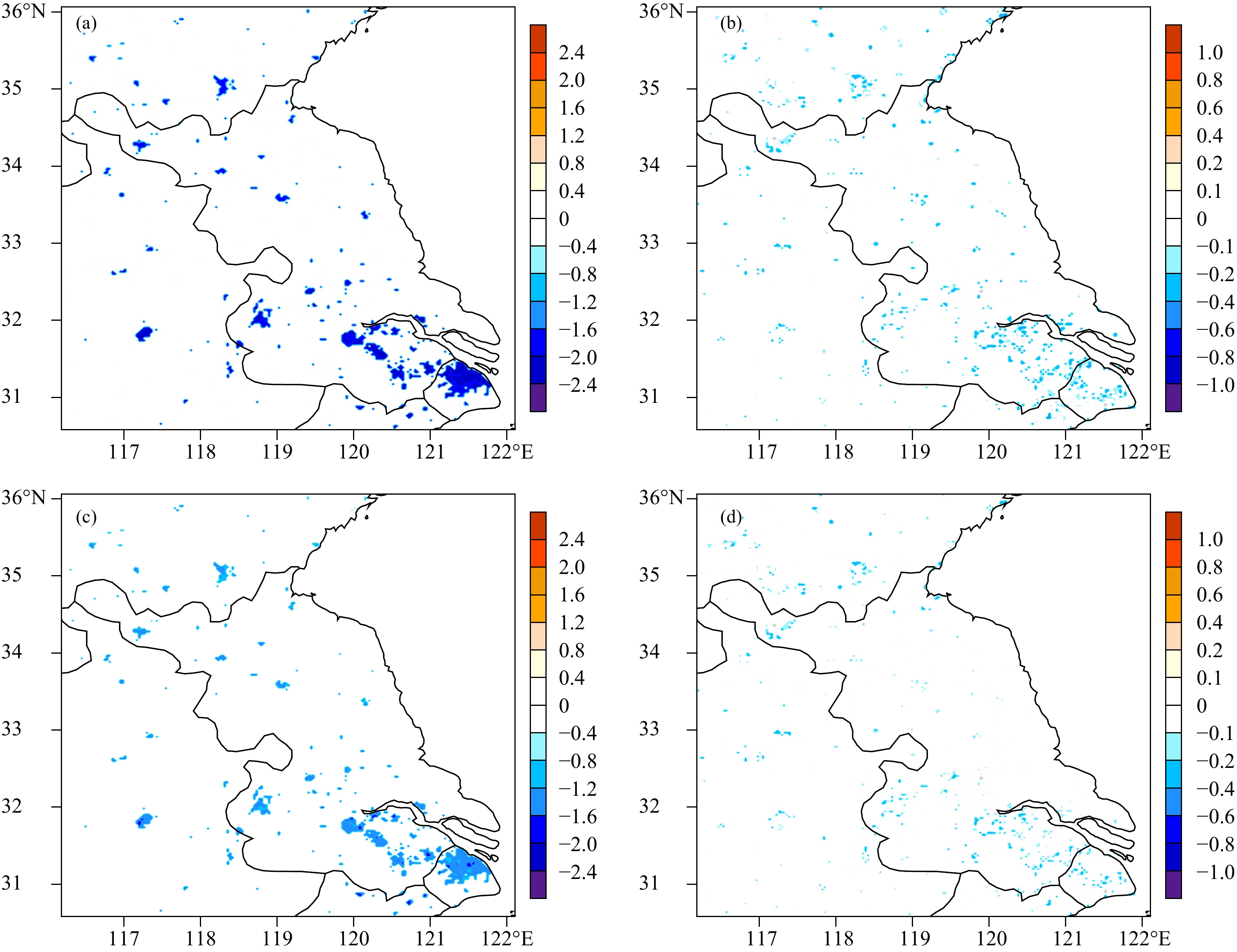图 11  光伏屋顶 （a、b. 支架式，c、d. 贴覆式） 与普通屋顶空调能耗 （单位：W/m2） 的差值 （a、c. 06—18时平均，b、d.19—05时平均） Fig. 11  Spatial distributions of the influence of photovoltaic roof （a，b. the bracket roof，c，d. the attached roof） on air conditioning energy consumption （a，c. the average cooling rates during 06:00—18:00 BT; b，d. the average cooling rates during 19:00—05:00 BT）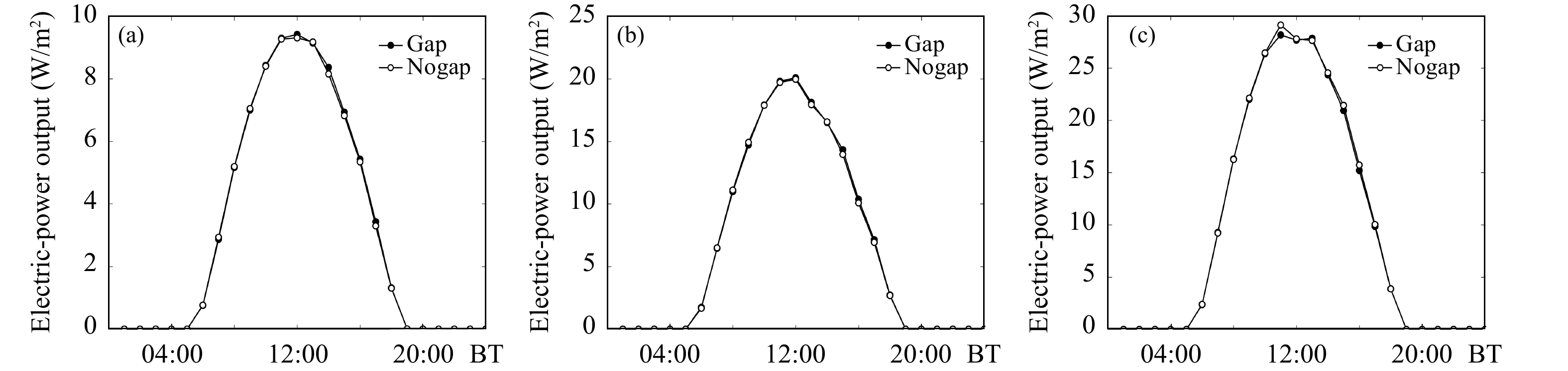图 12  光伏屋顶发电量的日变化特征 （a. 低密度住宅区，b. 高密度住宅区，c. 商业区） Fig. 12  Diurnal variation characteristics of photovoltaic roof power generation （a. low-density residential area，b. high-density residential area，c. commercial area）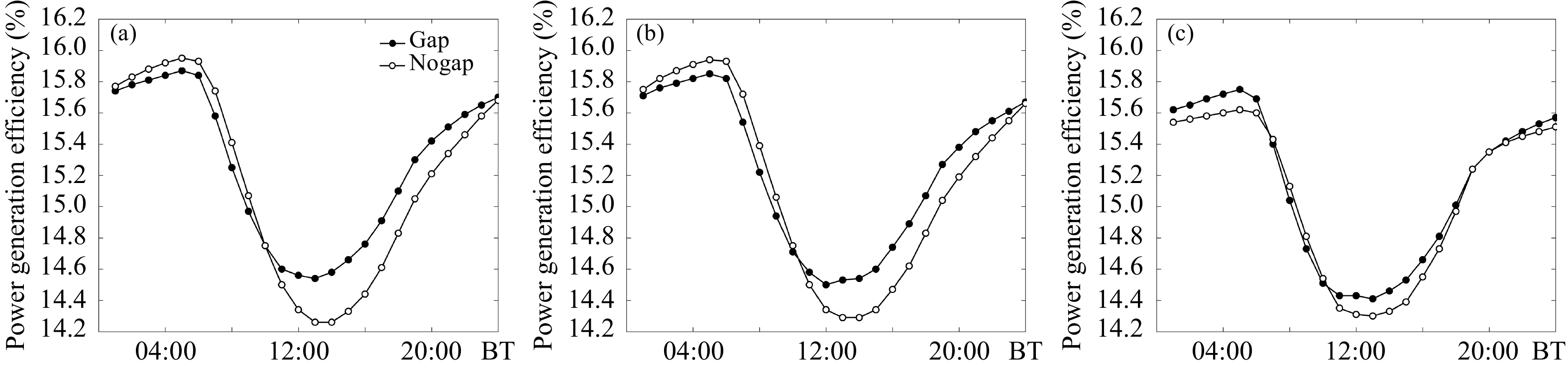图 13  光伏屋顶发电效率的日变化特征 （a. 低密度住宅区，b. 高密度住宅区，c. 商业区） Fig. 13  Diurnal variation characteristics of photovoltaic roof power generation efficiency （a. low-density residential area，b. high-density residential area，c. commercial area）
 $a = \frac{\,1\,}{\,4\,} \times P \times \left(\frac{{{B_{\rm w}} \times {B_{\rm l}}}}{{({S_{\rm w}} + {B_{\rm w}}) \times {B_{\rm l}}}}{\rm{ + }}\frac{{{B_{\rm w}} \times {B_{\rm l}}}}{{({S_{\rm l}} + {B_{\rm l}}) \times {B_{\rm w}}}}\right)$

 低密度住宅区 高密度住宅区 商业区 发电量（W/m2） 3.23 6.70 9.76 空调能耗（W/m2） 1.68 5.64 17.91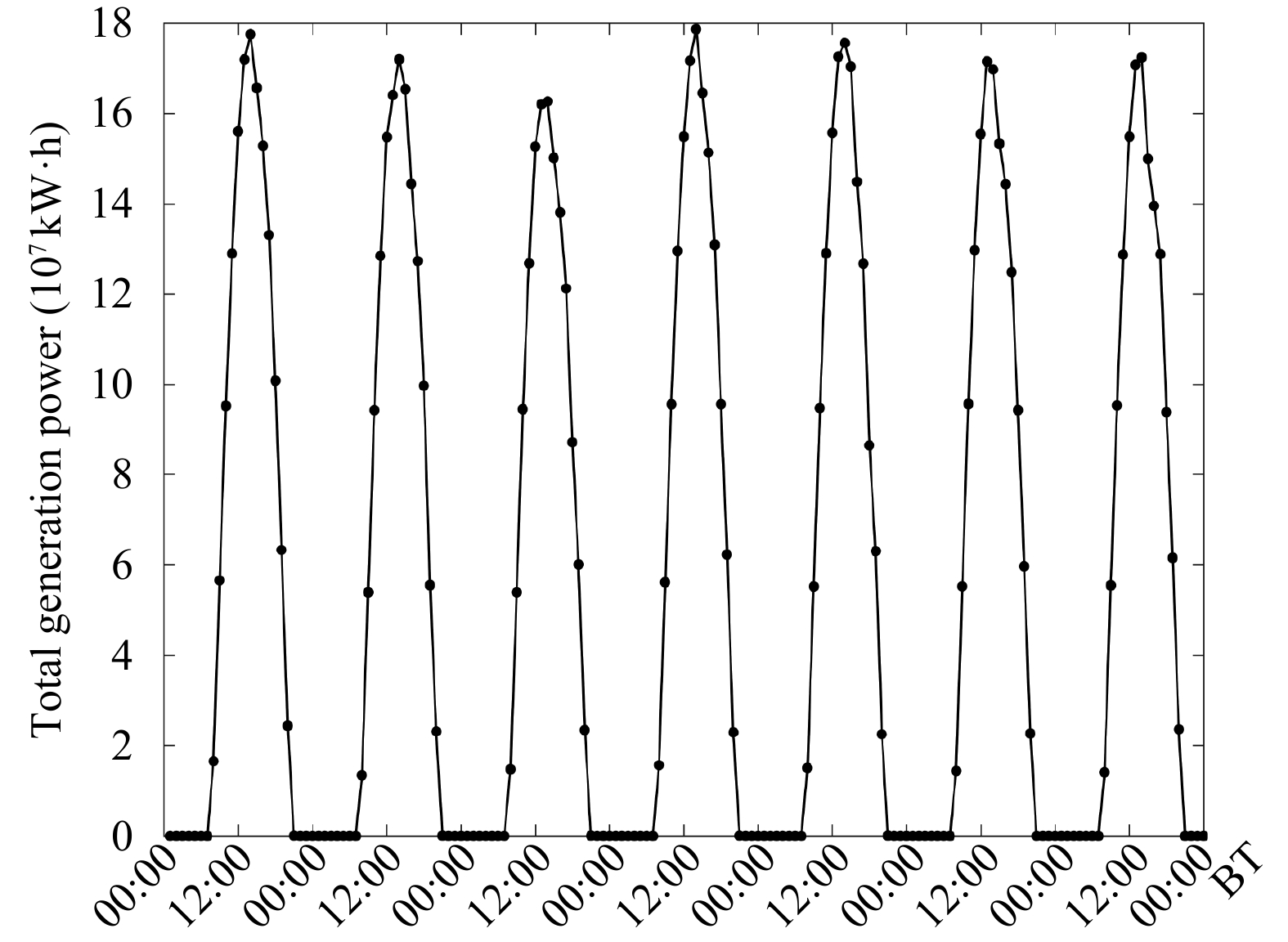图 14  全省光伏屋顶发电量逐时变化 Fig. 14  Hourly change of photovoltaic roof power generation in Jiangsu
4 结论和讨论

（1）2017年7月21—27日江苏省高温热浪期间，安装贴覆式光伏屋顶可以使城市2 m气温白天平均降低0.29°C，降温效果优于支架式屋顶，但夜间降温效果不明显。支架式屋顶白天降温0.23°C，夜间降温效果明显，最高降温0.60°C。

（2）光伏屋顶白天对城市确实可以起到降温作用，但同时也会削弱湍流动能，一定程度上抑制白天边界层的发展。在湍流发展旺盛的情形下，光伏屋顶的削弱作用更加明显。贴覆式屋顶由于白天表面温度更低，对大气的加热作用弱于其他两种屋顶，湍流动能强度最弱。

（3）光伏屋顶除了影响城市气象条件，最重要的还是对能源的贡献。文中将城市分作高密度、低密度居民区以及商业区3类进行讨论，从结果上看，当城市屋顶100%铺设太阳能光伏电池板时，住宅区光伏发电量基本能够满足同期用电需求，商业区发电量能够负担同期空调54.5%的能耗。商业区由于建筑分布密集，它的区域发电量高于其他两种城市类型，但是商业区高层建筑物比例较大，空调的能耗要远高于其他两种城市类型，承载能力弱。结合两种屋顶的模拟结果，贴覆式屋顶对城市热环境的降温效果更好，但对于室内空调能耗的节能效果弱于支架式屋顶。从城市节能角度看，使用支架式屋顶效果更加明显，但支架式屋顶的架设成本高于贴覆式屋顶，因此在城市建筑设计时，应综合考虑收益及成本，选取最适合的架设方式。

 艾欣, 韩晓男, 孙英云. 2013. 大型光伏电站并网特性及其低碳运行与控制技术. 电网技术, 37(1): 15-23. DOI:10.3969/j.issn.1674-0629.2013.01.004 李耀孙, 石春娥, 杨军等. 2012. 我国东部地区冬季模式边界层探空效果评估. 高原气象, 31(6): 1690-1703. 刘晓明. 2017. 基于能源结构视角下的江苏风电发展对策探究. 智库时代, (15): 210，213. 任建波. 2006. 光伏屋顶形式优化的实验和理论研究[D]. 天津: 天津大学. Ren J B. 2006. Experimental and theoretical study on photovoltaic roofs[D]. Tianjin: Tianjin University （in Chinese） 田玮. 2006. 光伏建筑的性能优化及其与城市微气候的相互影响[D]. 天津: 天津大学. Tian W. 2006. Performance optimization of building integrated photovoltaics and its interaction with urban microclimate[D]. Tianjin: Tianjin University （in Chinese） 王国复, 叶殿秀, 张颖娴等. 2018. 2017年我国区域性高温过程特征及异常大气环流成因分析. 气候变化研究进展, 14(4): 341-349. DOI:10.12006/j.issn.1673-1719.2018.008 王进华. 2007. 激光加工技术使太阳能电池的效率提高到22%. 光机电信息, 24(7): 23-24. DOI:10.3969/j.issn.1007-1180.2007.07.001 王玥. 2017. 光伏屋顶遮阳与供电综合节能研究[D]. 西安: 西安建筑科技大学. Wang Y. 2017. Study on comprehensive energy-saving of shading and photovoltaics of roof added PV module[D]. Xi'an: Xi'an University of Architecture and Technology （in Chinese） 张艳晴, 刘寿东, 王咏薇等. 2019. 南京地区太阳能屋顶缓解夏季高温的模拟研究. 气象学报, 77(2): 358-370. 张艳, 鲍文杰, 余琦等. 2012. 超大城市热岛效应的季节变化特征及其年际差异. 地球物理学报, 55(4): 1121-1128. DOI:10.6038/j.issn.0001-5733.2012.04.007 赵媛, 郝丽莎. 2006. 江苏省电力工业空间结构优化研究. 长江流域资源与环境, 15(3): 292-297. DOI:10.3969/j.issn.1004-8227.2006.03.005 Afonso C, Oliveira A. 2000. Solar chimneys: Simulation and experiment. Energy Build, 32(1): 71-79. DOI:10.1016/S0378-7788(99)00038-9 Atkinson B W. 2003. Numerical modelling of urban heat-island intensity. Bound Layer Meteor, 109(3): 285-310. DOI:10.1023/A:1025820326672 Chi W F, Shi W J, Kuang W H. 2015. Spatio-temporal characteristics of intra-urban land cover in the cities of China and USA from 1978 to 2010. J Geogr Sci, 25(1): 3-18. DOI:10.1007/s11442-015-1149-z Dominguez A, Kleissl J, Luvall J C. 2011. Effects of solar photovoltaic panels on roof heat transfer. Solar Energy, 85(9): 2244-2255. DOI:10.1016/j.solener.2011.06.010 Dudhia J. 1989. Numerical study of convection observed during the Winter Monsoon Experiment using a mesoscale two-dimensional model. J Atmos Sci, 46(20): 3077-3107. DOI:10.1175/1520-0469(1989)046<3077:NSOCOD>2.0.CO;2 Golden J S. 2006. Photovoltaic canopies: thermodynamics to achieve a sustainable systems approach to mitigate the urban heat island hysteresis lag effect. Int J Sustain Energy, 25(1): 1-21. DOI:10.1080/14786450600593139 Hansen J, Sato M, Ruedy R, et al. 2006. Global temperature change. Proc Natl Acad Sci USA, 103(39): 14288-14293. DOI:10.1073/pnas.0606291103 Hassid S, Santamouris M, Papanikolaou N, et al. 2000. The effect of the Athens heat island on air conditioning load. Energy Build, 32(2): 131-141. DOI:10.1016/S0378-7788(99)00045-6 Hong S Y, Lim J O J. 2006. The WRF single-moment 6-class microphysics scheme （WSM6）. J Korean Meteor Soc, 42(2): 129-151. Janjić Z I. 1994. The step-mountain eta coordinate model: Further developments of the convection, viscous sublayer, and turbulence closure schemes. Mon Wea Rev, 122(5): 927-945. DOI:10.1175/1520-0493(1994)122<0927:TSMECM>2.0.CO;2 Krauter S, Hanitsch R. 1990. The influence of the capsulation on the efficiency of PV-modules//Sayigh A A M. Energy and the Environment. Amsterdam: Elsevier, 371-375 Liu J Y, Kuang W H, Zhang Z X, et al. 2014. Spatiotemporal characteristics, patterns, and causes of land-use changes in China since the late 1980s. J Geogr Sci, 24(2): 195-210. DOI:10.1007/s11442-014-1082-6 Louis J F. 1979. A parametric model of vertical eddy fluxes in the atmosphere. Bound-Layer Meteor, 17(2): 187-202. DOI:10.1007/BF00117978 Masson V, Bonhomme M, Salagnac J L, et al. 2014. Solar panels reduce both global warming and urban heat island. Front Environ Sci, 2: 14. Mlawer E J, Taubman S J, Brown P D, et al. 1997. Radiative transfer for inhomogeneous atmospheres: RRTM, a validated correlated-k model for the longwave. J Geophys Res Atmos, 102(D14): 16663-16682. DOI:10.1029/97JD00237 Oke T R. 1987. Boundary Layer Climate. Cambridge: Great Britain at the University Press, 471-508 Sailor D J. 1995. Simulated urban climate response to modifications in surface albedo and vegetative cover. J Appl Meteor, 34(7): 1694-1704. DOI:10.1175/1520-0450-34.7.1694 Salamanca F, Martilli A. 2010. A new building energy model coupled with an urban canopy parameterization for urban climate simulations--part II. Validation with one dimension off-line simulations. Theor Appl Climatol, 99(3-4): 345-356. Salamanca F, Georgescu M, Mahalov A, et al. 2016. Citywide impacts of cool roof and rooftop solar photovoltaic deployment on near-surface air temperature and cooling energy demand. Bound Layer Meteor, 161(1): 203-221. DOI:10.1007/s10546-016-0160-y Tewari M, Chen F, Wang W, et al. 2004. Implementation and verification of the unified NOAH land surface model in the WRF model//Proceedings of the 20th Conference on Weather Analysis and Forecasting/16th Conference on Numerical Weather Prediction. Seattle: Amer Meteor Soc, 11-15 Tian W, Wang Y P, Xie Y Y, et al. 2007. Effect of building integrated photovoltaics on microclimate of urban canopy layer. Build Environ, 42(5): 1891-1901. DOI:10.1016/j.buildenv.2006.02.022 Xu X Y, Chen F, Shen S H, et al. 2018. Using WRF-urban to assess summertime air conditioning electric loads and their impacts on urban weather in Beijing. J Geophys Res Atmos, 123(5): 2475-2490. DOI:10.1002/2017JD028168 Yang H X, Zhu Z J, Burnett J, et al. 2001. A simulation study on the energy performance of photovoltaic roofs. ASHRAE Trans, 107(2): 129-135. Zhang N, Chen Y, Luo L, et al. 2017. Effectiveness of different urban heat island mitigation methods and their regional impacts. J Hydrometeorol, 18(11): 2991-3012. DOI:10.1175/JHM-D-17-0049.1